Tag: moduli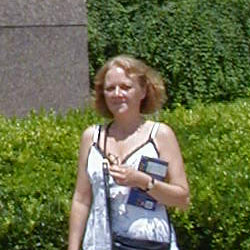This morning, Esther Beneish
arxived the paper The center of the generic algebra of degree p that may contain the most
significant advance in my favourite problem for over 15 years! In it she
claims to prove that the center of the generic division algebra of
degree p is stably rational for all prime values p. Let me begin by
briefly explaining what the problem is all about. Consider one n by n
matrix A which is sufficiently general, then it will have all its
eigenvalues distinct, but then it is via the Jordan normal form theorem uniquely
determined upto conjugation (that is, base change) by its
characteristic polynomial. In
other words, the conjugacy class of a sufficiently general n by n matrix
depends freely on the coefficients of the characteristic polynomial
(which are the n elementary symmetric functions in the eigenvalues of
the matrix). Now what about couples of n by n matrices (A,B) under
simultaneous conjugation (that is all couples of the form $~(g A g^{-1}, g B g^{-1})$ for some invertible n by n matrix g) ??? So,
does there exist a sort of Jordan normal form for couples of n by n
matrices which are sufficiently general? That is, are there a set of
invariants for such couples which determine it is freely upto
simultaneous conjugation?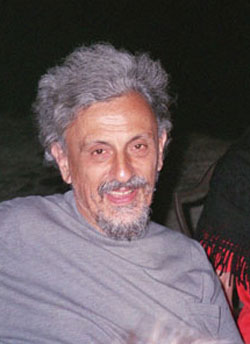For couples of 2 by 2 matrices, Claudio Procesi rediscovered an old
result due to James Sylvester saying
that this is indeed the case and that the set of invariants consists of
the five invariants Tr(A),Tr(B),Det(A),Det(B) and Tr(AB). Now, Claudio
did a lot more in his paper. He showed that if you could prove this for
couples of matrices, you can also do it for triples, quadruples even any
k-tuples of n by n matrices under simultaneous conjugation. He also
related this problem to the center of the generic division algebra of
degree n (which was introduced earlier by Shimshon Amitsur in a rather
cryptic manner and for a while he simply refused to believe Claudio’s
description of this division algebra as the one generated by two
_generic_ n by n matrices, that is matrices filled with independent
variables). Claudio also gave the description of the center of this
algebra as a field of lattice-invariants (over the symmetric group S(n)
) which was crucial in subsequent investigations. If you are interested
in the history of this problem, its connections with Brauer group
problems and invariant theory and a short description of the tricks used
in proving the results I’ll mention below, you might have a look at the
talk Centers of Generic Division Algebras, the rationality problem 1965-1990
I gave in Chicago in 1990.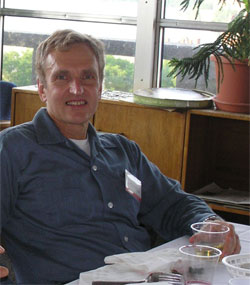The case of couples of 3 by 3 matrices was finally
settled in 1979 by Ed Formanek and a
year later he was able to solve also the case of couples of 4 by 4
matrices in a fabulous paper. In it, he used solvability of S(4) in an
essential way thereby hinting at the possibility that the problem might
no longer have an affirmative answer for larger values of n. When I read
his 4×4 paper I believed that someone able to prove such a result must
have an awesome insight in the inner workings of matrices and decided to
dedicate myself to this problem the moment I would get a permanent
job… . But even then it is a reckless thing to do. Spending all of
your time to such a difficult problem can be frustrating as there is no
guarantee you’ll ever write a paper. Sure, you can find translations of
the problem and as all good problems it will have connections with other
subjects such as moduli spaces of vectorbundles and of quiver
representations, but to do the ‘next number’ is another matter.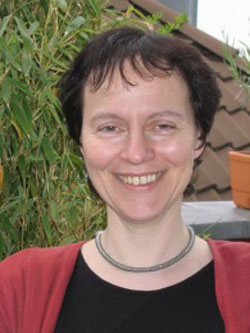Fortunately, early 1990, together with
Christine Bessenrodt we were
able to do the next two ‘prime cases’ : couples of 5 by 5 and couples of
7 by 7 matrices (Katsylo and Aidan Schofield had already proved that if
you could do it for couples of k by k and l by l matrices and if k and l
were coprime then you could also do it for couples of kl by kl matrices,
so the n=6 case was already done). Or did we? Well not quite, our
methods only allowed us to prove that the center is stably rational
that is, it becomes rational by freely adjoining extra variables. There
are examples known of stably rational fields which are NOT rational, but
I guess most experts believe that in the case of matrix-invariants
stable rationality will imply rationality. After this paper both
Christine and myself decided to do other things as we believed we had
reached the limits of what the lattice-method could do and we thought a
new idea was required to go further. If today’s paper by Esther turns
out to be correct, we were wrong. The next couple of days/weeks I’ll
have a go at her paper but as my lattice-tricks are pretty rusty this
may take longer than expected. Still, I see that in a couple of weeks
there will be a meeting in
Atlanta were Esther
and all experts in the field will be present (among them David Saltman
and Jean-Louis Colliot-Thelene) so we will know one way or the other
pretty soon. I sincerely hope Esther’s proof will stand the test as she
was the only one courageous enough to devote herself entirely to the
problem, regardless of slow progress.

Here are
my nominees for the 2006 paper of the year award in mathematics &
mathematical physics : in math.RA : math.RA/0606241
: Notes on A-infinity
algebras, A-infinity categories and non-commutative geometry. I
by

Maxim Kontsevich
and
Yan Soibelman
. Here is the abstract :

We develop
geometric approach to A-infinity algebras and A-infinity categories
based on the notion of formal scheme in the category of graded vector
spaces. Geometric approach clarifies several questions, e.g. the notion
of homological unit or A-infinity structure on A-infinity functors. We
discuss Hochschild complexes of A-infinity algebras from geometric point
of view. The paper contains homological versions of the notions of
properness and smoothness of projective varieties as well as the
non-commutative version of Hodge-to-de Rham degeneration conjecture. We
also discuss a generalization of Deligne’s conjecture which includes
both Hochschild chains and cochains. We conclude the paper with the
description of an action of the PROP of singular chains of the
topological PROP of 2-dimensional surfaces on the Hochschild chain
complex of an A-infinity algebra with the scalar product (this action is
more or less equivalent to the structure of 2-dimensional Topological
Field Theory associated with an “abstract” Calabi-Yau
manifold).

why ? : Because this paper
probably gives the correct geometric object associated to a
non-commutative algebra (a huge coalgebra) and consequently the right
definition of a map between noncommutative affine schemes. In a previous post (and its predecessors) I’ve
tried to explain how this links up with my own interpretation and since
another time. in hep-th : hep-th/0611082 : Children’s Drawings From
Seiberg-Witten Curves
by Sujay K. Ashok, Freddy Cachazo, Eleonora
Dell’Aquila. Here is the abstract :

We consider N=2
supersymmetric gauge theories perturbed by tree level superpotential
terms near isolated singular points in the Coulomb moduli space. We
identify the Seiberg-Witten curve at these points with polynomial
equations used to construct what Grothendieck called “dessins
d’enfants” or “children’s drawings” on the Riemann
sphere. From a mathematical point of view, the dessins are important
because the absolute Galois group Gal(\bar{Q}/Q) acts faithfully on
them. We argue that the relation between the dessins and Seiberg-Witten
theory is useful because gauge theory criteria used to distinguish
branches of N=1 vacua can lead to mathematical invariants that help to
distinguish dessins belonging to different Galois orbits. For instance,
we show that the confinement index defined in hep-th/0301006 is a Galois
invariant. We further make some conjectures on the relation between
Grothendieck’s programme of classifying dessins into Galois orbits and
the physics problem of classifying phases of N=1 gauge theories.

why ? : Because this paper gives the
best introduction I’ve seen to Grothendieck’s dessins d’enfants
(slightly overdoing it by giving a crash course on elementary Galois
theory in appendix A) and kept me thinking about dessins and their
Galois invariants ever since (again, I’ll come back to this later).

Three years ago I did spend three weeks next to my Canonscan, painstakingly scanning all individual pages of every preprint I ever wrote. Next, I converted every page to PDF, resized it (in order to control the size) and bundled them into PDF-files. A typical preprint would take me roughly three quarters of an hour and the final result was mediocre. For example, here a blown-up sample from the original 1992 ‘Moduli
spaces of right ideals of the Weyl algebra’ -preprint, resulting in a 1.7Mb PDF-file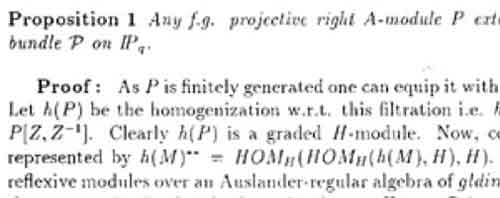Recentlty, the department bought a Ricoh-copier which makes scanning a lot more fun. To scan a preprint at 300dpi and convert it into a single PDF-file takes under a minute (actually, downloading the file using a web-interface takes longer…). For this particular preprint, the resulting PDF-file took up 1.2Mb and looks a lot nicer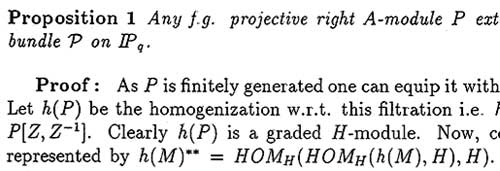Still, 1.2Mb is a huge file but converting it to a DjVu-file (DjVu=deja vu) using the handy Any2DjVu Service gives us a mere 236Kb file which comes a lot closer to the filesize of a PDFLaTeX-file and the output is still very legible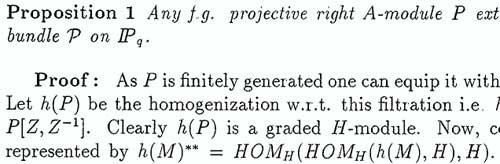So, I decided to rescan my entire life at 300dpi and convert it into DjVu. Next, I got the MOPP-package (MOPP = My Online Publications Page) working using the instructions from this page and some obvious MacOSX-modifications (if I can do it, so can you but perhaps I’ll write up the details in another post, just to remind myself). You can see the result at my homepage. I’ll update the latter one regularly (there are still some preprints missing, as are all my courses etc. and cross-references) and only afterwards I’ll update my homepage again. So far there is 250Mb to download (including all versions of the noncommutative geometry@n book, including the published ones…) so this should keep you busy for a while…

For
finite dimensional hereditary algebras, one can describe its
noncommutative topology (as developed in part 2)
explicitly, using results of Markus
Reineke
in The monoid
of families of quiver representations
. Consider a concrete example,
say

$A = \begin{bmatrix} \mathbb{C} & V \\ 0 & \mathbb{C} \end{bmatrix}$ where $V$ is an n-dimensional complex vectorspace, or
equivalently, A is the path algebra of the two point, n arrow quiver
$\xymatrix{\vtx{} \ar@/^/[r] \ar[r] \ar@/_/[r] & \vtx{}}$
Then, A has just 2 simple representations S and T (the vertex reps) of
dimension vectors s=(1,0) and t=(0,1). If w is a word in S and T we can
consider the set $\mathbf{r}_w$ of all A-representations having a
Jordan-Holder series with factors the terms in w (read from left to
right) so $\mathbf{r}_w \subset \mathbf{rep}_{(a,b)}~A$ when there are a
S-terms and b T-terms in w. Clearly all these subsets can be given the
structure of a monoid induced by concatenation of words, that is
$\mathbf{r}_w \star \mathbf{r}_{w’} = \mathbf{r}_{ww’}$ which is
Reineke’s *composition monoid*. In this case it is generated by
$\mathbf{r}_s$ and $\mathbf{r}_t$ and in the composition monoid the
following relations hold among these two generators
$\mathbf{r}_t^{\star n+1} \star \mathbf{r}_s = \mathbf{r}_t^{\star n} \star \mathbf{r}_s \star \mathbf{r}_t \quad \text{and} \quad \mathbf{r}_t \star \mathbf{r}_s^{\star n+1} = \mathbf{r}_s \star \mathbf{r}_t \star \mathbf{r}_s^{\star n}$ With these notations we can
now see that the left basic open set in the noncommutative topology
(associated to a noncommutative word w in S and T) is of the form
$\mathcal{O}^l_w = \bigcup_{w’} \mathbf{r}_{w’}$ where the union is
taken over all words w’ in S and T such that in the composition monoid
the relation holds $\mathbf{r}_{w’} = \mathbf{r}_w \star \mathbf{r}_{u}$
for another word u. Hence, each op these basic opens hits a large number
of $~\mathbf{rep}_{\alpha}$, in fact far too many for our purposes….
So, what do we want? We want to define a noncommutative notion of
birationality and clearly we want that if two algebras A and B are
birational that this is the same as saying that some open subsets of
their resp. $\mathbf{rep}$’s are homeomorphic. But, what do we
understand by *noncommutative birationality*? Clearly, if A and B are
prime Noethrian, this is clear. Both have a ring of fractions and we
demand them to be isomorphic (as in the commutative case). For this
special subclass the above noncommutative topology based on the Zariski
topology on the simples may be fine.

However, most qurves don’t have
a canonical ‘ring of fractions’. Usually they will have infinitely
many simple Artinian algebras which should be thought of as being
_a_ ring of fractions. For example, in the finite dimensional
example A above, if follows from Aidan Schofield‘s work Representations of rings over skew fields that
there is one such for every (a,b) with gcd(a,b)=1 and (a,b) satisfying
$a^2+b^2-n a b < 1$ (an indivisible Shur root for A).

And
what is the _noncommutative birationality result_ we are aiming
for in each of these cases? Well, the inspiration for this comes from
another result by Aidan (although it is not stated as such in the
paper…) Birational
classification of moduli spaces of representations of quivers
. In
this paper Aidan proves that if you take one of these indivisible Schur
roots (a,b) above, and if you look at $\alpha_n = n(a,b)$ that then the
moduli space of semi-stable quiver representations for this multiplied
dimension vector is birational to the quotient variety of
$1-(a^2+b^2-nab)$-tuples of $n \times n$-matrices under simultaneous
conjugation.

So, *morally speaking* this should be stated as the
fact that A is (along the ray determined by (a,b)) noncommutative
birational to the free algebra in $1-(a^2+b^2-nab)$ variables. And we
want a noncommutative topology on $\mathbf{rep}~A$ to encode all these
facts… As mentioned before, this can be done by replacing simples with
bricks (or if you want Schur representations) but that will have to wait
until next week.

Hectic
days ahead! Today, there is the Ph.D. defense of Stijn Symens and the
following two days there is a meeting in Ghent where Jacques
Alev and me organize a special session on non-commutative algebra. Here
is the programme of that section

Session 1 (Friday 20 May)
— chair : Jacques Alev (Univ. Reims)

15.30-16.25 : Iain Gordon (Glasgow, United
Kingdom) : “Rational Cherednik algebras and resolutions of
symplectic
singularities”

16.25-16.35 : break

16.35-17.30 : Olivier Schiffmann (ENS Paris, France) :
“Elliptic Hall algebras and spherical Cherednik algebras”

Session 2 (Saturday 21 May) — chair : Lieven Le Bruyn
(Univ. Antwerp)

14.30-15.15 : Markus
Reineke
(Munster, Germany) : “Geometry of Quiver Moduli”

15.15-16.00 : Raf Bocklandt &
Geert Van de Weyer
(Antwerp, Belgium) : “The power of slicing in noncommutative
geometry”

Afterwards it will be time to take a short
vacation (and do some cycling in the French mountains). Here is my
reading list for next week :

The dark Eye – Ingrid
Black